Next: The isentropic equations Up: Kinetic Formulations and Regularity Previous: Kinetic and other approximations

# Degenerate parabolic equations

As an example one can treat convective equations together with (possibly degenerate) diffusive terms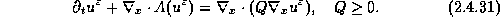Assume the problem is not linearly degenerate, in the sense that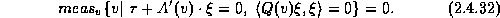Let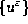be a family of approximate solutions of (2.2.7) with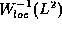-compact entropy production,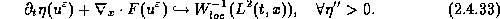Thenis compact in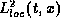, .

The case Q = 0 corresponds to our multidimensional discussion in §2.3.15; the case A =0 correspond possibly degenerate parabolic equations (consult  and the references therein, for example). According to (2.4.32), satisfying the ellipticity condition,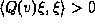on a set of non-zero measure, guarantees regularization, compactness ...

Again, a second-order version of the averaging lemmaenables us to quantify the gained regularity which we state as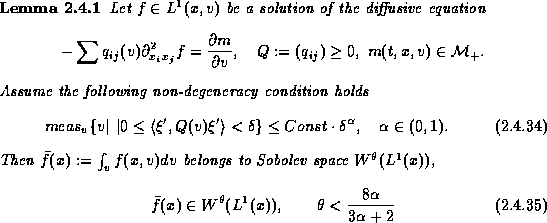Example. Consider the isotropic equation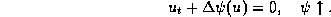Here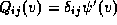and the lemma 2.4.1 applies. The kinetic formulation of such equations was studied in . In the particular case of porous media equation,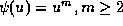, (2.4.34) holds with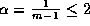and one conclude a regularizing effect of order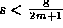, i.e.,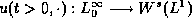.
A particular attractive advantage of the kinetic formulation in this case, is that it applies to non-isotropic problems as well.